# GMAT Math : Calculating the area of a square

## Example Questions

2 Next →

### Example Question #58 : Quadrilaterals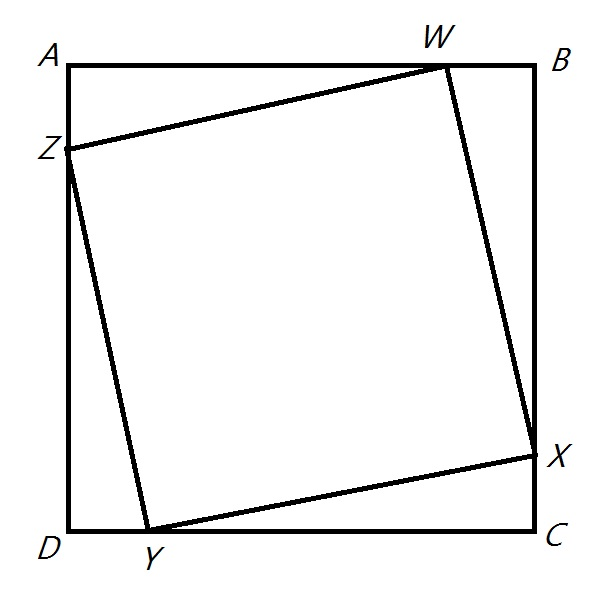Note: Figure NOT drawn to scale

Refer to the above figure, which shows Squareand Square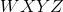. The ratio of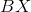to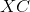is 13 to 2. What is the ratio of the area of Squareto that of Square?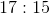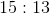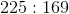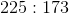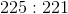Explanation:

To make this easier, assume that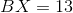and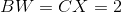- the reasoning generalizes. Then Squarehas sidelength 15 and area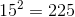. The sidelength of Square, each side being a hypotenuse of a right triangle with legs 2 and 13, is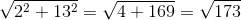.

The square of this, 173, is the area of Square.

The ratio is.

### Example Question #59 : QuadrilateralsNote: Figure NOT drawn to scale

Refer to the above figure, which shows Squareand Square.  The ratio oftois 7 to 1.

Which of these responses comes closest to what percent the area of Squareis of that of Square?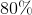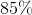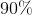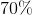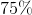Explanation:

To make this easier, assume that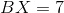and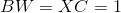; the results generalize.

Each side of Squarehas length 8, so the area of Squareis 64.

Each of the four right triangles has legs 7 and 1, so each has area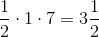; Squarehas area four times this subtracted from the area of Square, or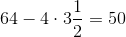.

The area of Squareis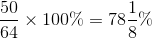of that of Square.

Of the five choices, 80% comes closest.

### Example Question #61 : Quadrilaterals

The perimeter of a square is the same as the circumference of a circle with area 100. What is the area of the square?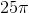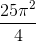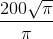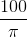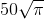Explanation:

The formula for the area of a circle is.

If the area is 100, the radius is as follows: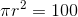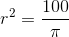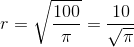The circle has circumferencetimes its radius, or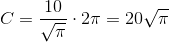This is also the perimeter of the square, so the sidelength of the square is one-fourth this, or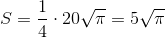The area of the square is the square of this, or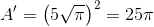### Example Question #62 : Quadrilaterals

The perimeter of a square is the same as the circumference of a circle with radius 8. What is the area of the square?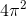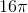The correct answer is not among the other choices.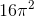Explanation:

A circle with radius 8 has as its circumferencetimes this, or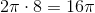.

This is also the perimeter of the square, so the sidelength is one fourth of this, or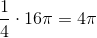.

The area is the square of this, or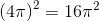.

### Example Question #63 : Quadrilaterals

The perimeter of a square is the same as the length of the hypotenuse of a right triangle with legs 8 and 12. What is the area of the square?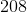The correct answer is not among the other responses.Explanation:

The length of the hypotenuse of a right triangle with legs 8 and 12 can be determined using the Pythagorean Theorem: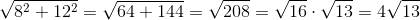Since this is also the perimeter of the square, its sidelength is one fourth of this, or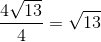The area of the square is the square of this sidelength, or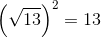### Example Question #21 : Squares

If the perimeter of a square is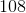, what is its area?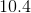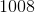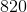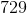Explanation:

The perimeter of a square, and any shape for that matter, is found by adding up all the exterior sides. Since all sides are equal in a square, we can say: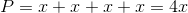whererepresents the length of a side

We can solve for the side length using the information provided: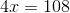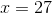The area of a square is found by squaring the side length: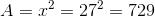### Example Question #65 : Quadrilaterals

The perimeter of a square is. Give its area.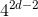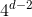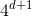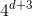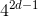Explanation:

The length of one side of a square is the perimeter divided by 4: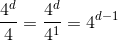Square this to get the area: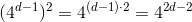2 Next →

Tired of practice problems?

Try live online GMAT prep today.